C++ Programming

# String Function: strtok, strcmp, strcpy, strlen, strstr, strcat, strcat in C++

String function- this header file contains the string related built-in functions. These functions are used to process strings. The most important and commonly used string functions of this header file are as described as under:

## Strlen() String Function:

The word ‘strlen’ stands for string length. The strlen() function is used to find the length of a string. This function counts the total number of characters in the string including spaces. The null character (‘\0’) is not counted.

The general syntax of this function is as follows:

Var = strlen(string);

Where

String: it indicates the given string whose length is to be found. It may be string variable or string constant.

Var: it indicates the integer variable into which the length of string is to be stored.

Top Gaming Computers

Best Laptops

Best Graphic Cards

Portable Hard Drives

Best Keyboards

Best High Quality PC Mic

Computer Accessories

### Programming example: write a program that inputs data into a string variable and finds its length using strlen string function:

```#include <iostream>
#include <string.h>
#include <stdio.h>

using namespace std;

int main()
{
char st;
cout<<" Enter any string :";
gets(st);
cout<<" Length of string is:"<<strlen(st);
}
```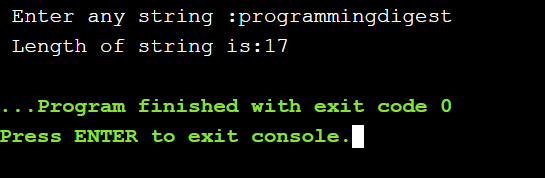## memcmp() String function:

the word ‘memcmp’ stand for memory compare. The memcmp() string function is use to compare specified number of bytes (or characters) of two string stored in two block of memory. Two string are compared byte-by-byte. The comparison is case sensitive.

The general syntax of this string function is as follows:

Var = memcmp(ptr1, ptr2, num);

Where

Ptr1: it represents pointer to first block of memory.

Ptr2: it represents pointer to second block of memory.

Num: it represents number of bytes to compare.

Var: it represents integer variable used to store the output of function.

This function returns output as follows:

• It returns 0 if first string is equal to second string.
• It returns less than 0 if first string is less than second string.
• It returns greater than 0 if first string is greater than second string.

### Programming example: write a program that compare two string using memcmp string function:

```#include <iostream>
#include <string.h>
#include <stdio.h>

using namespace std;

int main()
{
char *str1 ="abc";
char *str2 = "abc";
char *str3 = "xyz";
int n;
n=memcmp(str1, str2, 3);
if(n==0)
cout<<" Str1 is equal to Str2\n";
n=memcmp(str2, str3, 3);
if(n>0)
cout<<" str2 is greater than str3\n";
else
cout<<" str2 is less than str3\n";

}
```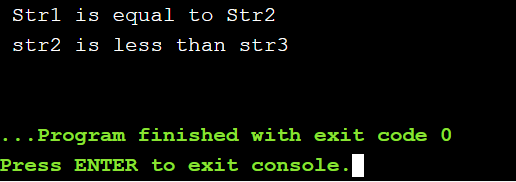## memicmp() String Function:

the ‘memicmp’ function is similar to ‘memcmp’ String function but here the comparison is not case-sensitive. It means that memicmp() String function ignores the character case( lower or upper). The letter ‘i’ in memicmp() function stands for ignore.

The general syntax of this function is as follows:

Var = memicmp(ptr1, ptr2, num);

For example, if:
s1= “abc”

s2= “ABC”

s3= “xyz”

memicmp(s1, s2, 3) will return 0

memicmp(s3, s2, 3) will return greater than 0

memicmp(s2, s3, 3) will return less than 0

## strcmp() String function:

the word ‘strcmp’ stands for string compare. The strcmp() function is used to compare two strings. The first string is compared with second string character by character. The comparison is case-sensitive.

The general syntax of this function is as follows:

Var = strcmp(string1, string2);

Where

String1: it represents the first string.

String2: it represents the second string.

Var: it represents the integer variable. It is used to store the result of comparison.

This function returns output as follows:

• Returns 0 if string1 is equal to string2.
• Returns less than 0 if string1 is less than string2.
• Returns greater than 0 if string1 is greater than string2.

For example, if:

S1=”abc”

S2=”abc”

S3=”xyz”

strcmp(S1, S2) will return 0.

strcmp(S3, S2) will return greater than 0.

strcmp(S2, S3) will return less than 0.

## stricmp() String Function:

the stricmp() string function is similar to strcmp() function. This function is also used to compare two string but the comparison is not case-sensitive. The first string is compared with second string character by character.

The general syntax of this function is as follows:

Var = stricmp(string1, string2);

Where

String1: it represents the first string.

String2: it represents the second string.

Var: it represents the integer variable. It is used to store the result of comparison.

This function returns output as follows:

• Returns 0 if string1 is equal to string2.
• Returns less than 0 if string1 is less than string2.
• Returns greater than 0 if string1 is greater than string2.

For example, if:

S1= “abc”

S2= “ABC”

S3= “xyz”

stricmp(S1, S2) will return 0.

stricmp(S3, S2) will return greater than 0.

stricmp(S2, S3) will return less than 0.

## strncmp() String function:

the strncmp() string function is similar to strcmp() function but it is used to compare the specified number of character of the two string.

The generl syntax of strncmp string function is as follows:

Var = strncmp(string1, string2, n);

Where

String1: it represents the first string.

String2: it represents the second string.

n: it represents a specified number of characters that are to be compared.

Var: it represents the integer variable. It is used to store the result of comparison.

This function returns output in similar way as strcmp() function.

For example, if:

S1=”abcdef”

S2= “abcdef”

S3=”xyzpqr”

strncmp(S1, S2, 3) will return 0.

strncmp(S3, S2, 3) will return greater than 0.

strncmp(S2, S3, 3) will return less than 0.

## strnicmp() String Function:

the strnicmp() string function is similar to stricmp() function but it is used to compare the specified number of characters of the two strings.

The general syntax of strnicmp() string function is as follows:

Var = stringcmp(string1, string2, n);

Where

String1: it represents the first string.

String2: it represents the second string.

n: it represents a specified number of characters that are to be compared.

Var: it represents the integer variable. It is used to store the result of comparison.

This function returns output in similar way as stricmp() function.

For example, if:

S1=”abcdef”

S2= “ABCDEF”

S3=”xyzpqr”

strnicmp (S1, S2, 3) will return 0.

strnicmp (S3, S2, 3) will return greater than 0.

strnicmp (S2, S3, 3) will return less than 0.

## strspn() String Function:

the strspn() sting function is used to determine the length of maximum initial part of a source string that consists only of characters in a specified list. If every character in the source string appears in the list, strnp() string function returns the length to the source string. If the first character in the source string is not in the list, strspn() function returns zero.

The general syntax of this function is as follows:

Var = strspn(string1, char_list);

Where

String1: it represents the source string.

Char_list: it represents the characters list.

Var: it represents integer type variable where result is to be stored.

### Programming example: write a program that determines the initial lengths of three strings using strspn string function:

```#include <iostream>
#include <string.h>
#include <stdio.h>

using namespace std;

int main()
{
int i;
char string1[]="123abc123";
char string2[]="123456";
char string3[]="a123456";
char char_list[] = "1234567890";
i=strspn(string1,char_list);
cout<<" the length of the initial number for string1 is : "<<i<<endl;
i=strspn(string2, char_list);
cout<<" the length of the initial number for string2 is : "<<i<<endl;
i= strspn(string3, char_list);
cout<<" the length of the initial number for string3 is : "<<i<<endl;

}
```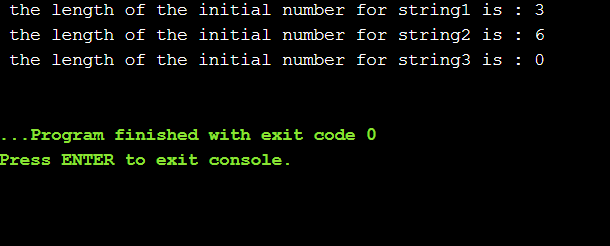In this program, the first three characters of string1 are digits, so strspn() function returns three. The string2 consists entirely of digits, so strspn() function returns the length of string2. Similarly, the first character of string3 is not a digits, so even though the rest of string3 consists of digits, strspn() function returns 0.

## strcspn() String Function:

the strcspn() string function is used to search a source string for the first occurrence of characters in a list and returns the number of characters read. This can include the terminating null character. So strcspn() function will return the length of the source string if it does not contain any of the characters for which it searches.

The general syntax of strcspn() string function is as following:
var = strcspn(string1, char_list);

Where

String1: it represents the source string.

Char_list: it represents the characters list.

Var: it represents integer type variable where result is to be stored.

### Programming example: write a program that explains the use of strcspn string function:

```#include <iostream>
#include <string.h>
#include <stdio.h>

using namespace std;

int main()
{
char string1[] = "abc2def";
char string2[] = "abcdef";
char char_list[] = "123456789012345";
int i;
i= strcspn(string1, char_list);
cout<<" the first digit 2 in string1 is at poistion :"<<i+1<<endl;
i=strcspn(string2, char_list);
cout<<" No character match, so lengh of string2 is :"<<i;

}
```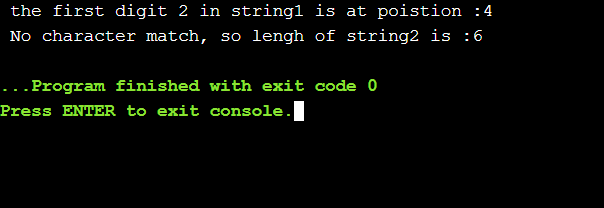## strcoll() String Function:

the strcoll() string function is used to compare two strings using collating sequence specified by setlocale() function. This function starts comparing the first character of each string. If they are equal to each other, this function continues with the following pair until the characters differ or until a null-character signaling the end of a string is reached.

The general syntax of this function is as follows:

strcoll(string1, string2);

where

string1: it represents first string.

String2: it represents second string.

This function returns output as follows:

• Returns 0 if string1 is equal to string2.
• Returns less than 0 if string1 is less than string2.
• Returns greater than 0 if string1 is greater than string2

## strstr() String Function:

the strstr() string function is used to find the first occurrence of a substring in another string. This function returns a pointer to the element in string where substring begins. It returns NULL if no occurrence found.

The general syntax of strstr() string function is as follows:

Var= strstr(string, substring);

Where

String: it represents a string.

Substring: it represents a substring.

Var: it represents pointer of char type where result is to be stored.4

For example, string “PROGRAMMING” is shown below with supposed memory addresses for each character.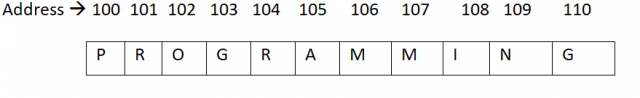The function strstr(“PROGRAMMIGN”,  “GRAM”) will return pointer to “G”(i.e. memory address 103)  because substring “GRAM” begins at this location.

### Programming example: write a program that explains the concept of strstr string function:

```#include <iostream>
#include <string.h>
#include <stdio.h>

using namespace std;

int main()
{
char st1[] = "programmingdigest";
char *pstr;
pstr = strstr(st1, "ing");
cout<<pstr<<endl;
cout<<pstr-st1<<endl;

}
```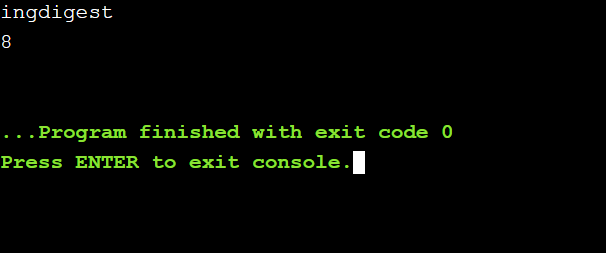In this program, the string “PROGRAMMING” is initialized to variable ‘st1’. The pointer variable “*pstr” is declared of char type to store the memory address from where the substring in string begins in memory. Suppose substring begins in string ‘st1’ at memory address 103. The statement “cout<<pstr<<endl;” will print the string from memory address 103 upto the end of string. So “GRAMMING”  will be printed.

Similarly, the statement “cout<<”pstr-st1<<endl;” will display 3. It is because the value of pstr and st1 are 103 and 100 (starting address of string ‘st1’) respectively. After performing subtraction operation, 3 will be displayed. This value also indicates that substring  begins after position 3 in the string.

## memchr() String function:

the word ‘memchr’ stands for memory character. The memchr() string function is used to search a specific character from a block of memory. It searches a specified number of bytes in a block of memory for the first occurrence of a specified character. It returns a pointer to this location and a null pointer if the value is not found.

The syntax of this function is as follows:

Void var = memchr(ptr, ch, size);

Where

Ptr: it indicates the pointer to the block of memory where search is performed. Name of string variable is given in this place.

Ch: it indicates the character to be searched from the buffer.

Size: it indicates the size of buffer in bytes.

Var: it indicates the pointer variable of type void.

### Programming example: write a program that searches a character from block of memory using memchr string function:

```#include <iostream>
#include <string.h>
#include <stdio.h>

using namespace std;

int main()
{
void *pch;
char *pos;
char *str = "C++ Programming";
pch = memchr(str,'g', strlen(str));
pos = (char *) pch;
if(pch !=NULL)
cout<<" \n Character 'g' found in string at position :"<<pos-str+1;
else

}
```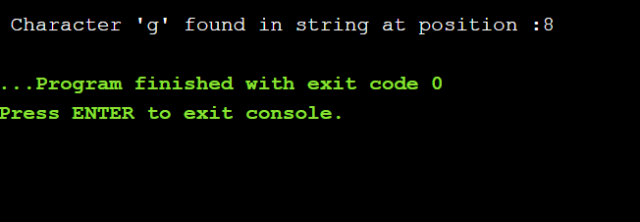## strchr() string function:

the strchr() string function is used to find the first occurrence of a given character in a string. This function returns a pointer to the element in the string where character occurs. It returns NULL if no occurrence found.

The general syntax of this function is as follows:

Var = strchr(string, character);

Where

String: it represents a string.

Character: it represents a give character to be found. It may be an ASCII value of a character.

Var: it represents pointer of char type where result is to be stored.

## strrchr() String Function:

the strrchr() string function is used to find the last occurrence of a given character in a string. This function returns a pointer to the element in the string where character occurs. It returns NULL if no occurrence found.

The general syntax of strrchr() string function is as follows:

Var = strrchr(string, character);

Where

String: it represents string in which the character is to be searched.

Character: it represents a given character to be found. It may be an ASCII value of a character.

Var: it represents pointer of char type where result is to be stored.

For example, string  “PROGRAMMING” is shown below with supposed memory addresses of each character.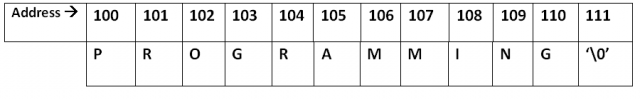The function strrchr(“PROGRAMMING”, ‘G’) will return pointer to the last occurrence of “A”, i.e. memory address 110.

## strpbrk() String Function:

the strpbrk() string function scans first string to find the first occurrence of any character that is in a second string. This function returns a pointer to the element in the string where character occurs. It returns NULL if no occurrence found.

The general syntax of strpbrk string function is as follows:

Var = strpbrk(string1, string2);

Where

String1: it represents first string.

String2: it represents second string.

Var: it represents pointer of char type where result is to be stored.

For example, string “programming” is shown below with supposed memory addresses of each character.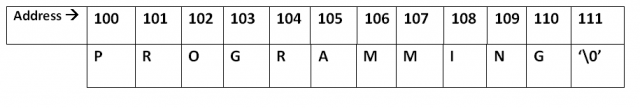The function strpbrk (“programming”, “mar”) will return pointer to “r”(i.e. memory address 101). It is because the first occurrence of character “r” in a second string is found in first string.

## Strrev() String function:

The sttrev() string function changes all characters  in a string to reverse order, except the null character. This string function returns a pointer to the reversed string.

The genera syntax of this function is as follows:

Var = strrev(string);

Where

String: it represent a string.

Var = it represents pointer of char type where address of resulting string is to be stored.

For example, string “ABCDEFGH” is shown below with supposed memory addresses of each character.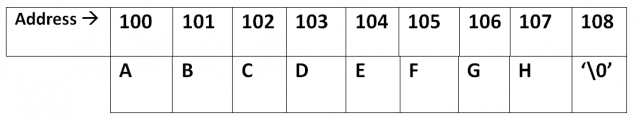After execution the function strrev(“ABCDEFGH”), result will be as under: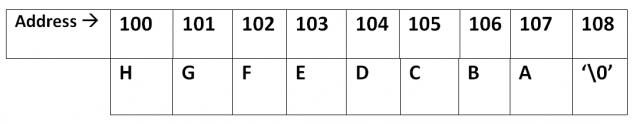## memset() String Function:

the memset() string function is use to fill specified size of block of memory with specified character. The general syntax of this function is as follows:

memset(prt, ch, size);

where

ptr: it represent pointer to the block of memory to fill.

Ch: it represents the character to be set in block of memory.

Size: it represent the size of memory block in bytes.

### Programming example: write a program that explains the use of memset string function:

```#include <iostream>
#include <string.h>
#include <stdio.h>

using namespace std;

int main()
{
char str[] = "Programmign with C++";
cout<<"str before applying memset() function\n";
cout<<"str :"<<str<<endl;
memset(str, '*', 8);
cout<<"str after applying memset() function\n";
cout<<"str: "<<str<<endl;

}
```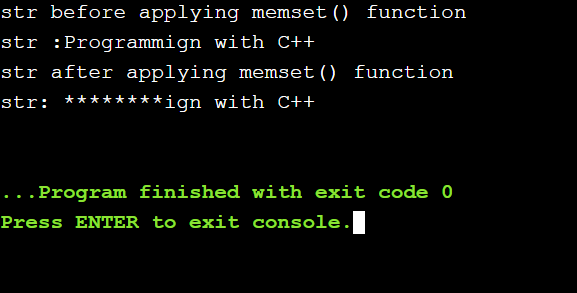## strset() String Function:

the strset() string function sets or replaces all characters( except null character) in a string with a specified character. The general syntax of this function is as follows:

strset(string, ch);

Where

String: it represents it string whose characters are to be replaced.

Ch: it represents the character to be used in place of each character of the string.

For example, string “ABCDEFGH” is show below.After executing the function strset(“ABCDEFGH”, ‘+’), result will be as under: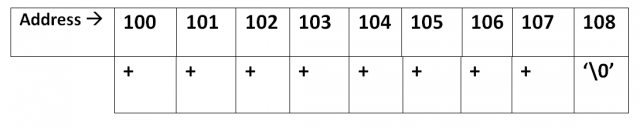## strnset() String Function:

the strnset() string function is used to set or replace specified number of characters at the beginning of string (except null character) with as specified character.

The general syntax of this function is as follows:

strnset(string, ch, n);

where

string: it represents the string whose characters are to be replaced.

Ch: it represents the character to be used in place of each character of the string.

n: it represents the number of character to be set.

For example, string “ABCDEFGH” is shown below.After executing the function strnset(“ABCDEFGH”, ‘*’, 4), the result will be as under: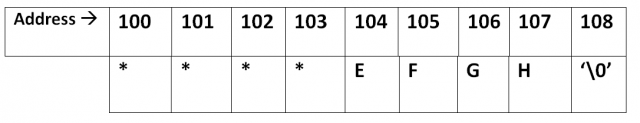## strlwr() string function:

the word ‘strlwr’ stands for string lowercase. The strlwr() string function is used to convert all uppercase letters(‘A’ to ‘Z’) of a string to lowercase letters. No other characters of the string are changed.

The general syntax of this function is as follows:

strlwr(string);

where

string: it represents the string whose characters are to be changed to lowercase.

For example, if string is “PROGRAMMING DIGEST”, then the function strlwr(string) will convert it “programming digest”.

## strupr() String function:

the word ‘strupr’ stands for string uppercase. The strupr() string function is used to convert all the lowercase letters(‘a’ to ‘z’) of a string to uppercase letters. No other characters of the string are changed.

The general syntax of this function is as follows:

strupr(string);

where

string: it represents the string whose characters are to be changed to lowercase.

For example, if string is “programming digest” then the function strupr(string) will convert it “PROGRAMMING DIGEST”.

## memcpy() string Function:

the word ‘memcpy’ stands for memory copy. The memcpy() string function is used to copy specified number of bytes (or characters) from on block of memory to another. It does not support overlapping.

The general syntax of this function is as follows:

memcpy(dest, src, num);

where

dest: it represents pointer to the destination array (block of memory) where the data or characters are to be copied.

Scr: it represents pointer to the source of data to be copied.

Num: it represents number of bytes or character to copy.

### Programming example: write a program that copies specified number of bytes from one block of memory to another using memcpy string function:

```#include <iostream>
#include <string.h>
#include <stdio.h>

using namespace std;

int main()
{
char str1[] = "simple string";
char str2, str3;
memcpy(str2, str1, strlen(str1)+1);
memcpy(str3, "programming with c++", 10);
cout<<" str1: "<<str1<<endl;
cout<<" str2: "<<str2<<endl;
cout<<" str3: "<<str3<<endl;

}
```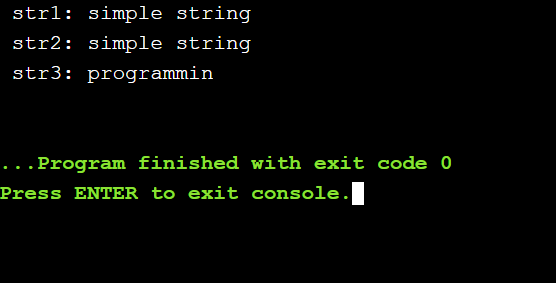## memmove() String Function:

the word ‘memmove’ stands for memory move. The memmove() string function is also used to copy specified number of bytes( or characters) from one block of memory to another. However, it supports overlapping. This function is also slower than memcpy() string function.

The general syntax of this function is as follows:

memmove(daest, src, num);

where

dest: it represents pointer to the destination array (block of memory) where the data or characters are to be copied.

Scr: it represents pointer to the source of data is to be copied.

Num: it represents number of bytes or character to copy.

## strcpy() string function:

the word ‘strcpy’ stands for string copy. The strcpy() function is used to copy one string to another string variable including null character(‘\0’).

The general syntax of this function is as follows:

strcpy(string1, string2);

where

string1: it represents the string variable where the contents of string2 are to be copied.

Stirng2: it represents the string whose contents are to be copied into string1. It may be a string constant or variable.

For example, to copy the string “OOP” to string variable ‘x’, the statement is written as:

strcpy(x, “OOP”);

### Programming example: write a program that exchanges the contents of one string to another using strcpy string function:

```#include <iostream>
#include <string.h>
#include <stdio.h>

using namespace std;

int main()
{
char st1[] = "strcpy";
char st2[] = "string";
char temp;
cout<<" contents of string before swapping\n";
cout<<" first string :"<<st1<<endl;
cout<<" second string :"<<st2<<endl;
strcpy(temp, st1);
strcpy(st1, st2);
strcpy(st2,temp);
cout<<" contents of string after swapping\n";
cout<<" first string :"<<st1<<endl;
cout<<" second string :"<<st2<<endl;

}
```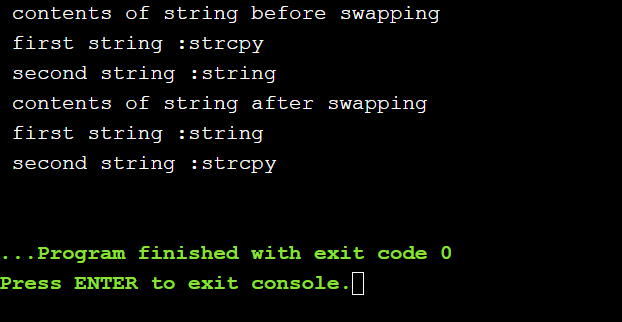## strncpy() String Function:

the strncpy() string function is used to copy a specified number of characters from one string to another string variable.

The general syntax of this function is as follows:

strncpy(string1, string2, n);

where

string1: it represents the string variable where the specified number of characters of string2 are to be copied. The null character (‘\0’) is not added at the end of string variable.

String2: it represents the string whose specified number of characters are to be copied into string1. It may be a string constant or variable.

n: it represents the number of characters of string2 that are to be copied into string1. It may be an integer constant or an integer type variable.

For example, to copy first three characters of string “Programming” in to the string variable ‘x’, the statement is written as:

strncpy(x, “Programming”, 3);

## strdup() String Function:

the word ‘strdup’ stands for string duplicate. The strdup() string function is used to make a duplicate copy of string into a new location in memory. This function returns the pointer to location that contains the duplicated string.

The general syntax of this function is as follows:

Var = strdup(string);

Where

String: it represents the string variable whose duplicate copy is to be created in memory.

Var: it represents the pointer of ‘char’ type where memory address of duplicated string is to be stored.

For example, to make the duplicate copy of string “Programming” into memory location and then to access the duplicated string, a set of statements is written as:

Char *ps, st1[]= “Programming”;

ps= strdup(st1);

cout<<ps;

## strcat() String Function:

the word ‘strcat’ stands for string concatenate. The strcat() string function is used to combine( or concatenate) the contents of one string to the end of another string variable. The null character is automatically added to the end of resulting string.

The general syntax of this function is as follows:

strcat(string1, string2);

where

string: it represents the string variable where the contents of string2 are to be added.

String2: it represents the string whose contents are to be added at end of string1. It may be a string constant or variable.

For example, to add the string “XYZ” into string variable x (which already contains “ABC”), the statement is written as:

strcat(x, “XYZ”);

after executing this function, the contents of variable ‘x’ will be “ABCXYZ”.

## strncat() String Function:

the strncat() string function is similar to strcat() string function but this function is used to combine (or concatenate) the specified number of characters of one string to the end of another string variable.

The general syntax of this function is as follows:

strncat(string1, string3, n);

where

string1: it represents the string variable where the specified number of characters of string2 are to be added.

String2: it represents the string whose specified number of characters are to be added at the end of string1. It may be a string constant or variable.

N: it represents the number of characters of string2 that are to be added at the end of string1.

For example, to add first three characters of string “programmingdigest” to string variable ‘x’, the statement is written as:

strncat(x, “programmingdigest”, 3);

## strtok() String Function:

the strtok() string function is used to split a string into tokens. It means that this function divides the string into smaller string based on the given character. A sequence of calls to this function split the string into tokens, which are sequences of contiguous characters separated by any of the characters that are part of delimiters.

The general syntax of this function is as follows;

strtok(string, delimiter);

where

string: it represents the string to be broken into tokens.

Delimiter: it represents the delimiter to be used for scanning.

### Programming example: write a program that splits the given strings using strtok string function:

```#include <iostream>
#include <string.h>
#include <stdio.h>

using namespace std;

int main()
{
char *str1 = "china, India, USA, Uk";
char *str2 = "Pakistan#Japan#Sweden#Turkey";
char * pch;
cout<<"splitting string1"<<endl;
pch=strtok(str1,",");
while(pch !=NULL)
{
cout<<pch<<endl;
pch = strtok(NULL, ",");

}
cout<<"\nSplitting string2"<<endl;
pch=strtok(str2,"#");
while(pch !=NULL)
{

cout<<pch<<endl;
pch=strtok(NULL,"#");
}
}
```